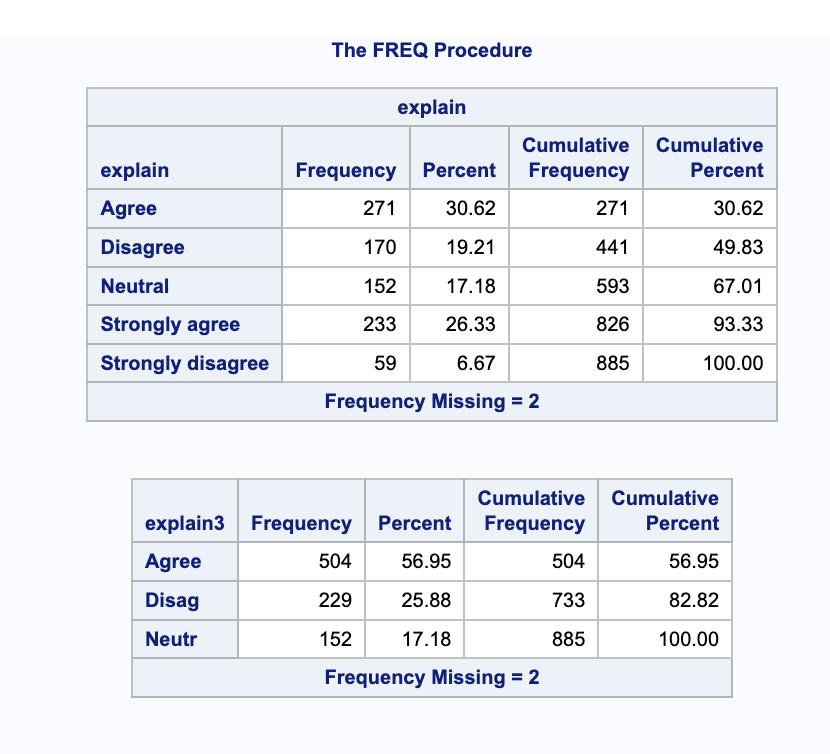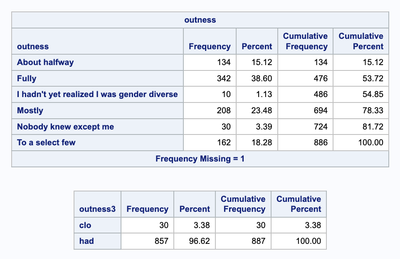## PROC FREQ cutting off value names at 5 characters, but only for new variables?

Today I tried to consolidate “strongly agree” with “agree” and “strongly disagree” with “disagree” for some of my variables. I was able to do that with if then statements, but afterwards, PROC FREQ incorrectly displayed value names, cutting them off after only five characters (but only for the new variables).

Also, when I initially tried to replace only two values and otherwise set the new variable equal to the old one, only the “strongly disagree” options changed. I'm having similar problems with a variable I had on a scale of six that I wanted to reduce to three.

Can anyone tell me what's wrong here?

``````Data GCvv;
SET GCv;
if explain = 'Agree' then explain3 = 'Agree';
if explain = 'Strongly agree' then explain3 = 'Agree';
if explain = 'Disagree' then explain3 = 'Disagree';
if explain = 'Strongly disagree' then explain3 = 'Disagree';
if explain = 'Neutral' then explain3 = 'Neutral';
RUN;

proc freq data=GCvv;
tables (explain explain3);
run;``````First code I tried:

``````Data GCvv;
SET GCv;
if explain = 'Strongly agree' then explain3 = 'Agree';
if explain = 'Strongly disagree' then explain3 = 'Disagree';
else explain3 = explain;
RUN;

proc freq data=GCvv;
tables (explain explain3);
run;``````Six options to three:

``````if outness = 'Mostly' then outness3 = 'out';
if outness = 'Fully' then outness3 = 'out';
if outness = 'About halfway' then outness3 = 'out';
if outness = 'To a select few' then outness3 = 'closeted';
if outness = 'Nobody knew except me' then outness3 = 'closeted';
else outness3 = 'had not realized';``````8 REPLIES 8

## Re: PROC FREQ cutting off value names at 5 characters, but only for new variables?

``````Data GCvv;
SET GCv;
length explain3 \$8.;
if explain = 'Agree' then explain3 = 'Agree';
if explain = 'Strongly agree' then explain3 = 'Agree';
if explain = 'Disagree' then explain3 = 'Disagree';
if explain = 'Strongly disagree' then explain3 = 'Disagree';
if explain = 'Neutral' then explain3 = 'Neutral';
RUN;

proc freq data=GCvv;
tables (explain explain3);
run;``````

Issue is in the data step, not FREQ.

Add the length statement to explicitly set the length of the new variable to 8 characters, otherwise it takes the length of the first value set to it ('Agree', 5 characters) by default.

@akimme wrote:

Today I tried to consolidate “strongly agree” with “agree” and “strongly disagree” with “disagree” for some of my variables. I was able to do that with if then statements, but afterwards, PROC FREQ incorrectly displayed value names, cutting them off after only five characters (but only for the new variables).

Also, when I initially tried to replace only two values and otherwise set the new variable equal to the old one, only the “strongly disagree” options changed.

Can anyone tell me what's wrong here?

``````Data GCvv;
SET GCv;
if explain = 'Agree' then explain3 = 'Agree';
if explain = 'Strongly agree' then explain3 = 'Agree';
if explain = 'Disagree' then explain3 = 'Disagree';
if explain = 'Strongly disagree' then explain3 = 'Disagree';
if explain = 'Neutral' then explain3 = 'Neutral';
RUN;

proc freq data=GCvv;
tables (explain explain3);
run;``````First code I tried:

``````Data GCvv;
SET GCv;
if explain = 'Strongly agree' then explain3 = 'Agree';
if explain = 'Strongly disagree' then explain3 = 'Disagree';
else explain3 = explain;
RUN;

proc freq data=GCvv;
tables (explain explain3);
run;``````## Re: PROC FREQ cutting off value names at 5 characters, but only for new variables?

Ohh okay, thanks, I didn't realize that was a separate step.

Do you know why I'm having the other problem, where SAS doesn't seem to be reading some of my if/then statements at all? I already checked to make sure I wasn't changing, eg, "strongly agree" to "agree" and then the next line changed "agree" to something else.

## Re: PROC FREQ cutting off value names at 5 characters, but only for new variables?

@akimme wrote:
Ohh okay, thanks, I didn't realize that was a separate step.

Do you know why I'm having the other problem, where SAS doesn't seem to be reading some of my if/then statements at all? I already checked to make sure I wasn't changing, eg, "strongly agree" to "agree" and then the next line changed "agree" to something else.

You would have to share some actual values and all the code that is used as it is not impossible that the issues may be related to other code that you think is working.

And as a completely different option you may want to consider custom formats. That way you can DISPLAY values without changing the values or having issues with if/then/else logic. If the values you want to use for analysis, reporting or graphing are based on a single variable formats are the most flexible and often the easiest way to address such. Below is an example of using a 1 to 5 numeric scale and a couple of demonstrations. Formats can also be applied to character variable just takes more typing.

```data demo;
do i=1 to 30;
x = rand('integer',5);
y = rand('integer',5);
output;
end;
run;

proc format;
value fivepointscale
1='Strongly Disagree'
2='Disagree'
3='Neutral'
4='Agree'
5='Strongly Agree'
;
value threepointscale
1,2 = 'Disagree'
3   = 'Neutral'
4,5 = 'Agree'
;
run;

proc freq data=demo;
title 'Use of the Five point scale';
tables x y;
format x y fivepointscale. ;
run;
proc freq data=demo;
title 'Use of the Three point scale';
tables x y;
format x y threepointscale. ;
run; title;```

What the above does. The first data set makes some observations with random values of 1 to 5 to have something to work with.

Proc format creates two different but similar display formats for the numeric ranges as shown. The five point scale is to show the "default" or original values. Then the three point consolidates them similar to your program adding new variables.

Then there are two calls to proc freq using the different formats for the same variables.

If you want to make character based formats then the name, the text after VALUE in Proc Format, starts with \$ to tell SAS it is using characters. Do not end the name of a format with a digit as that will generate an error.

This is even more efficient the more similar variables that you have. If you have 25 variables with the same responses then you only need 1 format to display the given text and can be applied to multiple variables as shown above. Saves adding 25 (or more) variables. This approach also allows, if desirable, some formats that maybe just combine the Disagree but leave two agree levels, or vice versa, to apply to specific variables. Or maybe have Disagree and Neutral grouped together. 0r even Strongly Disagree/Agree as two categories and combine the "somewhat" and neutral into a somewhat larger "middle ground" response.

You may note that the order of appearance in Proc freq is a bit different as well. That happens because the order, unless using an Order option, is to use the underlying value of the numeric variables and then applies the format for display. So the appearance order is in numeric order and you don't get Agree, Disagree, Neutral, Somewhat Agree and Somewhat Disagree order in the output.

If values are numeric and have ranges of interest, such as AGE, then it is easy to make different age range formats for reporting. I typically have about 10 related to ages because of different reporting requirements, 3-year, 5-year, 10-year intervals, specific over/under an age, think qualified/ not qualified for XXXX activity.

## Re: PROC FREQ cutting off value names at 5 characters, but only for new variables?

This code and the output below seem correct to me.

``````Data GCvv;
SET GCv;
if explain = 'Agree' then explain3 = 'Agree';
if explain = 'Strongly agree' then explain3 = 'Agree';
if explain = 'Disagree' then explain3 = 'Disagree';
if explain = 'Strongly disagree' then explain3 = 'Disagree';
if explain = 'Neutral' then explain3 = 'Neutral';
RUN;

proc freq data=GCvv;
tables (explain explain3);
run;``````

The counts in the output appear to match up correctly. What makes you think it's not working?

One way to check your coding that's slightly easier to see is to do a two way table:

``````proc freq data=GCvv;
tables explain*explain3 / MISSING;
run;``````

I would also recommend using IF/ELSE IF rather than multiple IF statements.

``````if explain = ...;
else if explain = ....;
else if ...``````

@akimme wrote:
Ohh okay, thanks, I didn't realize that was a separate step.

Do you know why I'm having the other problem, where SAS doesn't seem to be reading some of my if/then statements at all? I already checked to make sure I wasn't changing, eg, "strongly agree" to "agree" and then the next line changed "agree" to something else.

## Re: PROC FREQ cutting off value names at 5 characters, but only for new variables?

Using ELSE IF instead of just IF fixed another problem! Thank you

## Re: PROC FREQ cutting off value names at 5 characters, but only for new variables?

The reason the two "strongly" categories seem to disappear is most likely because the actual values are not what they look like in the ODS printout out showed.  ODS output has a VERY NASTY habit of removing leading spaces.  So that the first place I would loo.

But if could also be that the space between the two words is not actually a space but is instead some other invisible character.  Perhaps the character 'A0'x which some encoding consider a non-breaking space.

## Re: PROC FREQ cutting off value names at 5 characters, but only for new variables?

Ohh, okay, maybe if I copy and paste the names the characters will all match?

Thanks!

## Re: PROC FREQ cutting off value names at 5 characters, but only for new variables?

@akimme wrote:
Ohh, okay, maybe if I copy and paste the names the characters will all match?

Thanks!

If the problem is actually extra characters you are better off modifying the output.

And where you copy from is important as most of the output will not show them. Run this example and examine the results:

```data example;
length word \$ 10;
word='word';output;
word=' word';output;
word='  word';output;
word='   word';output;
run;

proc print data=example;
run;

proc freq data=example;
run;```

The data is build with differing numbers of leading spaces . Print strips them for output. Proc freq counts the different values with the leading spaces but then displays all of the results without the spaces. This is one way to determine that is an issue.

If the issue is a simple leading space a basic fix: The strip function will remove all leading spaces.

```data example2;
set example;
word= strip(word);
run;```

IF you run proc freq and still get apparent duplicate values then you have other characters appearing in your values and have to use different methods, such as putting the values with \$hex format. This will lead you into learning bits about ASCII or EBCDIC data character storage.

From SAS Users blog